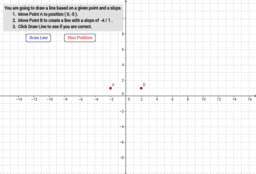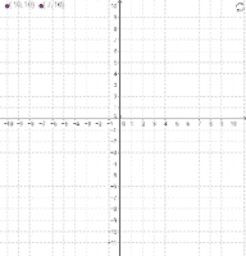# Linear Equations

Graphing equations using charts and y-intercept formula; solving 1-and 2-step equations
•### Linear Relationship: Graphing Lines Using a Point and Slope

•### Graph the Line

•